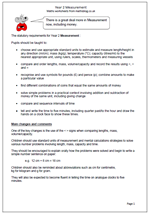# New Maths Curriculum: Year 2 MeasurementThe statutory requirements for Year 2 Measurement :

Pupils should be taught to:

•    choose and use appropriate standard units to estimate and measure length/height in any direction (m/cm); mass (kg/g); temperature (°C); capacity (litres/ml) to the nearest appropriate unit, using rulers, scales, thermometers and measuring vessels

•    compare and order lengths, mass, volume/capacity and record the results using >, < and =

•    recognise and use symbols for pounds (£) and pence (p); combine amounts to make a particular value

•    find different combinations of coins that equal the same amounts of money

•    solve simple problems in a practical context involving addition and subtraction of money of the same unit, including giving change

•    compare and sequence intervals of time

•    tell and write the time to five minutes, including quarter past/to the hour and draw the hands on a clock face to show these times.

One of the key changes is the use of the < > signs when comparing lengths, mass, volume/capacity.

Children should use standard units of measurement and mental calculations strategies to solve various number problems involving length, mass, capacity and time.

They should be encouraged to explain orally how the problems were solved and begin to write a simple number sentence on paper:

e.g.  12 cm + 6 cm = 18 cm

Children should also be reminded about abbreviations such as cm for centimetre,
kg for kilogram and g for gram.

They will also be expected to become fluent in telling the time on analogue clocks to five minutes.

New Maths Curriculum: Year 2 Measurement